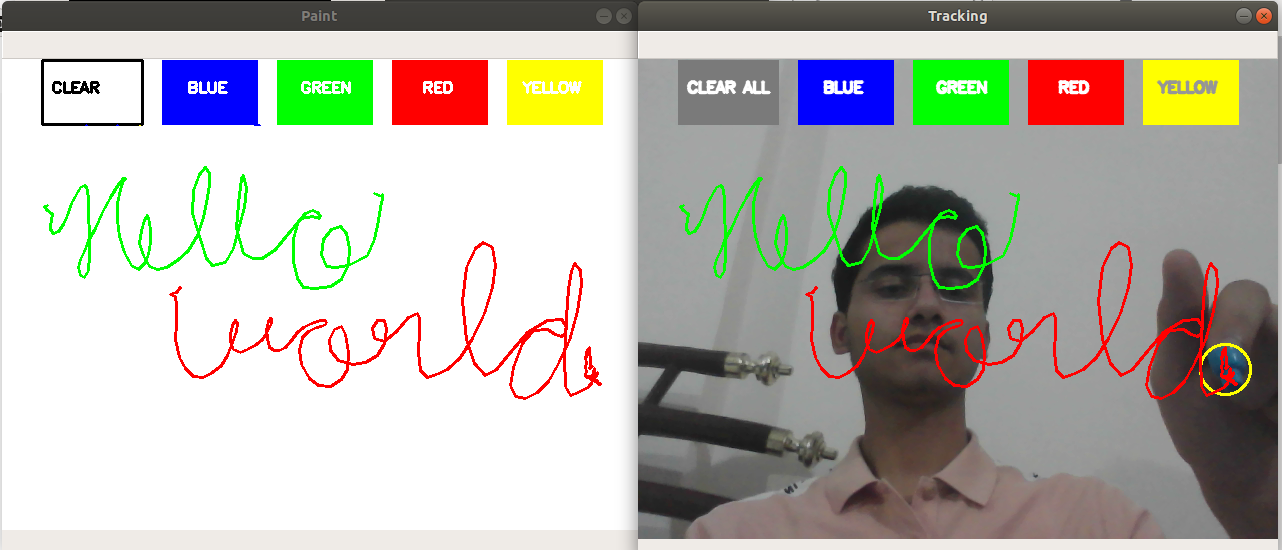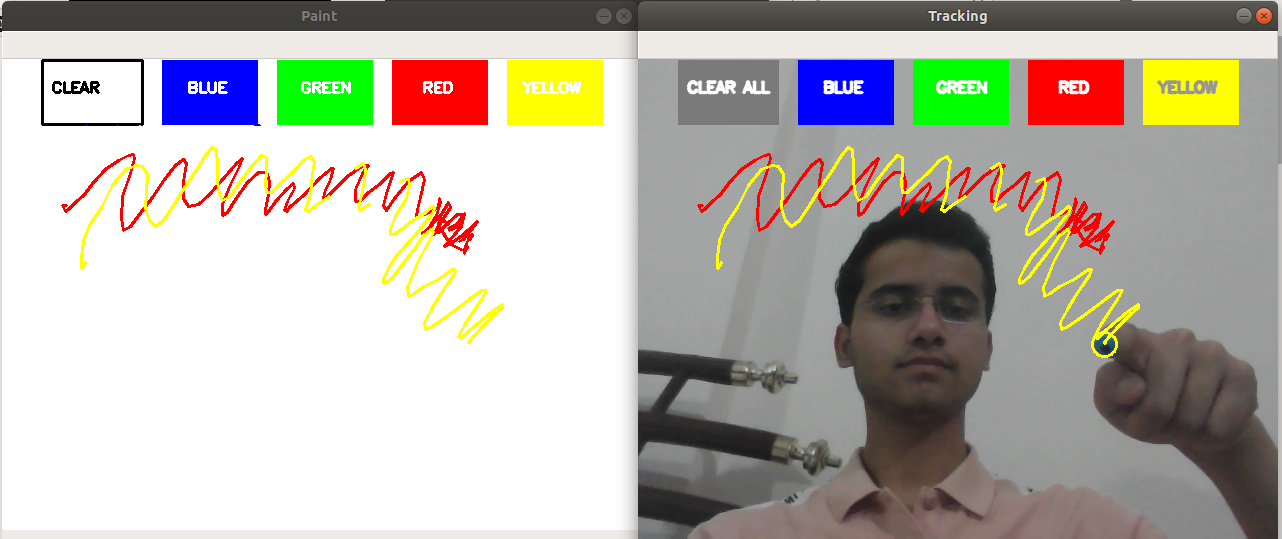Related Articles
Create Air Canvas using Python-OpenCV
• Difficulty Level : Medium
• Last Updated : 10 May, 2020

Ever wanted to draw your imagination by just waving your finger in the air. In this post, we will learn to build an Air Canvas which can draw anything on it by just capturing the motion of a colored marker with a camera. Here a colored object at the tip of the finger is used as the marker.

We will be using the computer vision techniques of OpenCV to build this project. The preferred language is Python due to its exhaustive libraries and easy to use syntax but understanding the basics it can be implemented in any OpenCV supported language.

Here Color Detection and tracking are used in order to achieve the objective. The color marker is detected and a mask is produced. It includes the further steps of morphological operations on the mask produced which are Erosion and Dilation. Erosion reduces the impurities present in the mask and dilation further restores the eroded main mask.

Requirements:

Algorithm:

1. Start reading the frames and convert the captured frames to HSV color space (Easy for color detection).
2. Prepare the canvas frame and put the respective ink buttons on it.
3. Adjust the track bar values for finding the mask of the colored marker.
4. Preprocess the mask with morphological operations (Eroding and dilation).
5. Detect the contours, find the center coordinates of largest contour and keep storing them in the array for successive frames (Arrays for drawing points on canvas).
6. Finally draw the points stored in an array on the frames and canvas.

Below is the implementation.

 `import` `numpy as np``import` `cv2``from` `collections ``import` `deque`` ` `  ` `# default called trackbar function ``def` `setValues(x):``   ``print``("")``  ` ` ` `# Creating the trackbars needed for ``# adjusting the marker colour These ``# trackbars will be used for setting ``# the upper and lower ranges of the``# HSV required for particular colour``cv2.namedWindow(``"Color detectors"``)``cv2.createTrackbar(``"Upper Hue"``, ``"Color detectors"``,``                   ``153``, ``180``, setValues)``cv2.createTrackbar(``"Upper Saturation"``, ``"Color detectors"``,``                   ``255``, ``255``, setValues)``cv2.createTrackbar(``"Upper Value"``, ``"Color detectors"``, ``                   ``255``, ``255``, setValues)``cv2.createTrackbar(``"Lower Hue"``, ``"Color detectors"``,``                   ``64``, ``180``, setValues)``cv2.createTrackbar(``"Lower Saturation"``, ``"Color detectors"``, ``                   ``72``, ``255``, setValues)``cv2.createTrackbar(``"Lower Value"``, ``"Color detectors"``, ``                   ``49``, ``255``, setValues)`` ` ` ` `# Giving different arrays to handle colour``# points of different colour These arrays ``# will hold the points of a particular colour``# in the array which will further be used``# to draw on canvas``bpoints ``=` `[deque(maxlen ``=` `1024``)]``gpoints ``=` `[deque(maxlen ``=` `1024``)]``rpoints ``=` `[deque(maxlen ``=` `1024``)]``ypoints ``=` `[deque(maxlen ``=` `1024``)]``  ` `# These indexes will be used to mark position``# of pointers in colour array``blue_index ``=` `0``green_index ``=` `0``red_index ``=` `0``yellow_index ``=` `0``  ` `# The kernel to be used for dilation purpose ``kernel ``=` `np.ones((``5``, ``5``), np.uint8)`` ` `# The colours which will be used as ink for``# the drawing purpose``colors ``=` `[(``255``, ``0``, ``0``), (``0``, ``255``, ``0``), ``          ``(``0``, ``0``, ``255``), (``0``, ``255``, ``255``)]``colorIndex ``=` `0``  ` `# Here is code for Canvas setup``paintWindow ``=` `np.zeros((``471``, ``636``, ``3``)) ``+` `255``  ` `cv2.namedWindow(``'Paint'``, cv2.WINDOW_AUTOSIZE)``  ` ` ` `# Loading the default webcam of PC.``cap ``=` `cv2.VideoCapture(``0``)``  ` `# Keep looping``while` `True``:``     ` `    ``# Reading the frame from the camera``    ``ret, frame ``=` `cap.read()``     ` `    ``# Flipping the frame to see same side of yours``    ``frame ``=` `cv2.flip(frame, ``1``)``    ``hsv ``=` `cv2.cvtColor(frame, cv2.COLOR_BGR2HSV)``  ` `    ``# Getting the updated positions of the trackbar``    ``# and setting the HSV values``    ``u_hue ``=` `cv2.getTrackbarPos(``"Upper Hue"``,``                               ``"Color detectors"``)``    ``u_saturation ``=` `cv2.getTrackbarPos(``"Upper Saturation"``,``                                      ``"Color detectors"``)``    ``u_value ``=` `cv2.getTrackbarPos(``"Upper Value"``,``                                 ``"Color detectors"``)``    ``l_hue ``=` `cv2.getTrackbarPos(``"Lower Hue"``,``                               ``"Color detectors"``)``    ``l_saturation ``=` `cv2.getTrackbarPos(``"Lower Saturation"``,``                                      ``"Color detectors"``)``    ``l_value ``=` `cv2.getTrackbarPos(``"Lower Value"``,``                                 ``"Color detectors"``)``    ``Upper_hsv ``=` `np.array([u_hue, u_saturation, u_value])``    ``Lower_hsv ``=` `np.array([l_hue, l_saturation, l_value])``  ` `  ` `    ``# Adding the colour buttons to the live frame ``    ``# for colour access``    ``frame ``=` `cv2.rectangle(frame, (``40``, ``1``), (``140``, ``65``), ``                          ``(``122``, ``122``, ``122``), ``-``1``)``    ``frame ``=` `cv2.rectangle(frame, (``160``, ``1``), (``255``, ``65``),``                          ``colors[``0``], ``-``1``)``    ``frame ``=` `cv2.rectangle(frame, (``275``, ``1``), (``370``, ``65``), ``                          ``colors[``1``], ``-``1``)``    ``frame ``=` `cv2.rectangle(frame, (``390``, ``1``), (``485``, ``65``), ``                          ``colors[``2``], ``-``1``)``    ``frame ``=` `cv2.rectangle(frame, (``505``, ``1``), (``600``, ``65``),``                          ``colors[``3``], ``-``1``)``     ` `    ``cv2.putText(frame, ``"CLEAR ALL"``, (``49``, ``33``),``                ``cv2.FONT_HERSHEY_SIMPLEX, ``0.5``,``                ``(``255``, ``255``, ``255``), ``2``, cv2.LINE_AA)``     ` `    ``cv2.putText(frame, ``"BLUE"``, (``185``, ``33``), ``                ``cv2.FONT_HERSHEY_SIMPLEX, ``0.5``,``                ``(``255``, ``255``, ``255``), ``2``, cv2.LINE_AA)``     ` `    ``cv2.putText(frame, ``"GREEN"``, (``298``, ``33``),``                ``cv2.FONT_HERSHEY_SIMPLEX, ``0.5``,``                ``(``255``, ``255``, ``255``), ``2``, cv2.LINE_AA)``     ` `    ``cv2.putText(frame, ``"RED"``, (``420``, ``33``),``                ``cv2.FONT_HERSHEY_SIMPLEX, ``0.5``, ``                ``(``255``, ``255``, ``255``), ``2``, cv2.LINE_AA)``     ` `    ``cv2.putText(frame, ``"YELLOW"``, (``520``, ``33``),``                ``cv2.FONT_HERSHEY_SIMPLEX, ``0.5``, ``                ``(``150``, ``150``, ``150``), ``2``, cv2.LINE_AA)``  ` `  ` `    ``# Identifying the pointer by making its ``    ``# mask``    ``Mask ``=` `cv2.inRange(hsv, Lower_hsv, Upper_hsv)``    ``Mask ``=` `cv2.erode(Mask, kernel, iterations ``=` `1``)``    ``Mask ``=` `cv2.morphologyEx(Mask, cv2.MORPH_OPEN, kernel)``    ``Mask ``=` `cv2.dilate(Mask, kernel, iterations ``=` `1``)``  ` `    ``# Find contours for the pointer after ``    ``# idetifying it``    ``cnts, _ ``=` `cv2.findContours(Mask.copy(), cv2.RETR_EXTERNAL,``        ``cv2.CHAIN_APPROX_SIMPLE)``    ``center ``=` `None``  ` `    ``# Ifthe contours are formed``    ``if` `len``(cnts) > ``0``:``         ` `        ``# sorting the contours to find biggest ``        ``cnt ``=` `sorted``(cnts, key ``=` `cv2.contourArea, reverse ``=` `True``)[``0``]``         ` `        ``# Get the radius of the enclosing circle ``        ``# around the found contour``        ``((x, y), radius) ``=` `cv2.minEnclosingCircle(cnt)``         ` `        ``# Draw the circle around the contour``        ``cv2.circle(frame, (``int``(x), ``int``(y)), ``int``(radius), (``0``, ``255``, ``255``), ``2``)``         ` `        ``# Calculating the center of the detected contour``        ``M ``=` `cv2.moments(cnt)``        ``center ``=` `(``int``(M[``'m10'``] ``/` `M[``'m00'``]), ``int``(M[``'m01'``] ``/` `M[``'m00'``]))``  ` `        ``# Now checking if the user wants to click on ``        ``# any button above the screen ``        ``if` `center[``1``] <``=` `65``:``             ` `            ``# Clear Button``            ``if` `40` `<``=` `center[``0``] <``=` `140``: ``                ``bpoints ``=` `[deque(maxlen ``=` `512``)]``                ``gpoints ``=` `[deque(maxlen ``=` `512``)]``                ``rpoints ``=` `[deque(maxlen ``=` `512``)]``                ``ypoints ``=` `[deque(maxlen ``=` `512``)]``  ` `                ``blue_index ``=` `0``                ``green_index ``=` `0``                ``red_index ``=` `0``                ``yellow_index ``=` `0``  ` `                ``paintWindow[``67``:, :, :] ``=` `255``            ``elif` `160` `<``=` `center[``0``] <``=` `255``:``                    ``colorIndex ``=` `0` `# Blue``            ``elif` `275` `<``=` `center[``0``] <``=` `370``:``                    ``colorIndex ``=` `1` `# Green``            ``elif` `390` `<``=` `center[``0``] <``=` `485``:``                    ``colorIndex ``=` `2` `# Red``            ``elif` `505` `<``=` `center[``0``] <``=` `600``:``                    ``colorIndex ``=` `3` `# Yellow``        ``else` `:``            ``if` `colorIndex ``=``=` `0``:``                ``bpoints[blue_index].appendleft(center)``            ``elif` `colorIndex ``=``=` `1``:``                ``gpoints[green_index].appendleft(center)``            ``elif` `colorIndex ``=``=` `2``:``                ``rpoints[red_index].appendleft(center)``            ``elif` `colorIndex ``=``=` `3``:``                ``ypoints[yellow_index].appendleft(center)``                 ` `    ``# Append the next deques when nothing is ``    ``# detected to avois messing up``    ``else``:``        ``bpoints.append(deque(maxlen ``=` `512``))``        ``blue_index ``+``=` `1``        ``gpoints.append(deque(maxlen ``=` `512``))``        ``green_index ``+``=` `1``        ``rpoints.append(deque(maxlen ``=` `512``))``        ``red_index ``+``=` `1``        ``ypoints.append(deque(maxlen ``=` `512``))``        ``yellow_index ``+``=` `1``  ` `    ``# Draw lines of all the colors on the``    ``# canvas and frame ``    ``points ``=` `[bpoints, gpoints, rpoints, ypoints]``    ``for` `i ``in` `range``(``len``(points)):``         ` `        ``for` `j ``in` `range``(``len``(points[i])):``             ` `            ``for` `k ``in` `range``(``1``, ``len``(points[i][j])):``                 ` `                ``if` `points[i][j][k ``-` `1``] ``is` `None` `or` `points[i][j][k] ``is` `None``:``                    ``continue``                     ` `                ``cv2.line(frame, points[i][j][k ``-` `1``], points[i][j][k], colors[i], ``2``)``                ``cv2.line(paintWindow, points[i][j][k ``-` `1``], points[i][j][k], colors[i], ``2``)``  ` `    ``# Show all the windows``    ``cv2.imshow(``"Tracking"``, frame)``    ``cv2.imshow(``"Paint"``, paintWindow)``    ``cv2.imshow(``"mask"``, Mask)``  ` `    ``# If the 'q' key is pressed then stop the application ``    ``if` `cv2.waitKey(``1``) & ``0xFF` `=``=` `ord``(``"q"``):``        ``break`` ` `# Release the camera and all resources``cap.release()``cv2.destroyAllWindows()`

Output:Attention geek! Strengthen your foundations with the Python Programming Foundation Course and learn the basics.

To begin with, your interview preparations Enhance your Data Structures concepts with the Python DS Course. And to begin with your Machine Learning Journey, join the Machine Learning – Basic Level Course

My Personal Notes arrow_drop_up